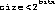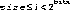`Packages  This Package  Prev  Next  Index  `

# §2.6 Class IndexColorModel

```public  class  java.awt.image.IndexColorModel
extends  java.awt.image.ColorModel  (II-§2.1)
{
// Constructors
public IndexColorModel(int  bits, int  size,	§2.6.1
byte  r[], byte  g[], byte  b[]);
public IndexColorModel(int  bits, int  size,	§2.6.2
byte  r[], byte  g[],
byte  b[], byte  a[]);
public IndexColorModel(int  bits, int  size,	§2.6.3
byte  r[], byte  g[],
byte  b[], int  trans);
public IndexColorModel(int  bits, int  size,	§2.6.4
byte  cmap[], int  start,
boolean  hasalpha);
public IndexColorModel(int  bits, int  size,	§2.6.5
byte  cmap[], int  start,
boolean  hasalpha,  int  trans);

// Methods
public final int getAlpha(int  pixel);	§2.6.6
public final void getAlphas(byte  a[]);	§2.6.7
public final int getBlue(int  pixel);	§2.6.8
public final void getBlues(byte  b[]);	§2.6.9
public final int getGreen(int  pixel);	§2.6.10
public final void getGreens(byte  g[]);	§2.6.11
public final int getMapSize();	§2.6.12
public final int getRed(int  pixel);	§2.6.13
public final void getReds(byte  r[]);	§2.6.14
public final int getRGB(int  pixel);	§2.6.15
public final int getTransparentPixel();	§2.6.16
}
```
The index color model class specifies a color model (II-§2.1) in a which a pixel value is converted into alpha, red, green, and blue component by using the pixel value as an index into a color map. Each entry in the color map gives the alpha, red, green, and blue component for the corresponding pixel.

An optional transparent pixel can be specified. This pixel is completely transparent, independent of the alpha value recorded for that pixel value.

The maximum size of the color map is 256 entries.

This color model is similar to an X11 PseudoColor visual.

Many of the methods in this class are final: the underlying native graphics code makes assumptions about the layout and operation of this class and those assumptions are reflected in the implementations of the methods here that are marked final. Applications can subclass this class for other reaons, but they cannot override or modify the behavior of the final methods.

##### IndexColorModel

```public IndexColorModel(int bits, int size, byte r[], byte g[], byte b[]) ```
Constructs an IndexColorModel from the given arrays of red, green, and blue components.
Pixels described by this color model all have alpha components of 255 (fully opaque).
Each of the three arrays must have at least size elements, and it must be the case that. These first size elements of the arrays are the red, green, and blue values for pixels in the range. Pixels in the rangehave red, green, and blue values of 0.
Parameters:
```bits - ```The number of bits in a pixel
```size - ```The size of the color component arrays
```r - ```an array of red color components
```g - ```an array of green color components
```b - ```an array of blue color components

### IndexColorModel

```public IndexColorModel(int bits, int size, byte r[], byte g[], byte b[], byte a[]) ```
Constructs an IndexColorModel from the given arrays of red, green, blue and alpha components.
Each of the four arrays must have at least size elements, and it must be the case that. These first size elements of the arrays are the red, green, and blue values for pixels in the range. Pixels in the rangehave red, green, and blue, and alpha values of 0.
Parameters:
```bits - ```The number of bits in a pixel
```size - ```a lower bound on the size of each of the arrays
```r - ```an array of red color components
```g - ```an array of green color components
```b - ```an array of blue color components
```a - ```an array of alpha value components

### IndexColorModel

```public IndexColorModel(int bits, int size, byte r[], byte g[], byte b[], int trans) ```
Constructs an IndexColorModel from the given arrays of red, green, and blue components.
Pixels described by this color model all have alpha components of 255 (fully opaque), except for the transparent pixel.
Each of the three arrays must have at least size elements, and it must be the case that. These first size elements of the arrays are the red, green, and blue values for pixels in the range. Pixels in the rangehave red, green, and blue values of 0.
Parameters:
```bits - ```The number of bits in a pixel
```size - ```The size of the color component arrays
```r - ```an array of red color components
```g - ```an array of green color components
```b - ```an array of blue color components
```trans - ```the index of the transparent pixel

### IndexColorModel

```public IndexColorModel(int bits, int size, byte cmap[], int start, boolean hasalpha) ```
Constructs an index color model from a single array of packed red, green, blue and optional alpha components.
If the hasalpha argument is false, then the cmap array must have length of at least. The red, green, and blue components for a pixel i in the rangeare in the three elements of the cmap array starting at cmap[start + 3i] Its alpha component is 255 (fully opaque).
If the hasalpha argument is true, then the cmap array must have length of at least. The red, green, blue, and alpha components for a pixel i in the rangeare in the four elements of the cmap array starting at cmap[start + 4i]
Pixels in the rangehave red, green, and blue values of 0, Their alpha component is 0 if hasalpha is true, and 255 otherwise.
Parameters:
```bits - ```the number of bits in a pixel
```size - ```the size of the color component arrays
```cmap - ```an array of color components
```start - ```the starting offset of the first color component
```hasalpha - ```if true, the alpha values are contained in the cmap array

### IndexColorModel

```public IndexColorModel(int bits, int size, byte cmap[], int start, boolean hasalpha, int trans) ```
Constructs an index color model from a single array of packed red, green, blue, and optional alpha values.
The color model is constructed the same way as described in §2.6.4. In addition, the specified transparent index represents a pixel which is considered entirely transparent regardless of any alpha value specified for it. The array must have enough values in it to fill all of the needed component arrays of the specified size.

Parameters:
```bits - ```the number of bits in a pixel
```size - ```the size of the color component arrays
```cmap - ```an array of color components
```start - ```the starting offset of the first color component
```hasalpha - ```if true, the alpha values are in the cmap array
```trans - ```The index of the fully transparent pixel.

##### getAlpha

```public final int getAlpha(int pixel) ```
Determines the alpha transparency value of the pixel in this color model. The value ranges from 0 to 255. The value 0 indicates that the pixel is completely transparent. The value 255 indicates that the pixel is opaque.
Parameters:
```pixel - ```a pixel value
Returns:
The alpha transparency represented by the pixel value
Overrides:
getAlpha in class ColorModel (II-§2.1.3).

### getAlphas

```public final void getAlphas(byte a[]) ```
Copies the array of alpha transparency values from this color model into the given array.
Only the initial entries of the array as specified by the getMapSize method (II-§2.6.12) are written.

Parameters:
```a - ```an array into which to place the results

### getBlue

```public final int getBlue(int pixel) ```
Determines the blue component of the pixel in this color model. The value ranges from 0 to 255. The value 0 indicates no contribution from this primary color. The value 255 indicates the maximum intensity of this color component.
Parameters:
```pixel - ```a pixel value
Returns:
the blue color component represented by the pixel value.
Overrides:
getBlue in class ColorModel (II-§2.1.4).

### getBlues

```public final void getBlues(byte b[]) ```
Copies the array of blue color components from this color model into the given array.
Only the initial entries of the array as specified by the getMapSize method (II-§2.6.12) are written.

Parameters:
```b - ```an array into which to place the results

### getGreen

```public final int getGreen(int pixel) ```
Determines the green component of the pixel in this color model. The value ranges from 0 to 255. The value 0 indicates no contribution from this primary color. The value 255 indicates the maximum intensity of this color component.
Parameters:
```pixel - ```a pixel value
Returns:
the blue color component represented by the pixel value.
Overrides:
getGreen in class ColorModel (II-§2.1.5).

### getGreens

```public final void getGreens(byte g[]) ```
Copies the array of green color components from this color model into the given array.
Only the initial entries of the array as specified by the getMapSize method (II-§2.6.12) are written.

Parameters:
```g - ```an array into which to place the results

### getMapSize

```public final int getMapSize() ```
Returns:
the value of the size argument when creating this index color model.

### getRed

```public final int getRed(int pixel) ```
Determines the red component of the pixel in this color model. The value ranges from 0 to 255. The value 0 indicates no contribution from this primary color. The value 255 indicates the maximum intensity of this color component.
Parameters:
```pixel - ```a pixel value
Returns:
the red color component represented by the pixel value.
Overrides:
getRed in class ColorModel (II-§2.1.7).

### getReds

```public final void getReds(byte r[]) ```
Copies the array of red color components from this color model into the given array.
Only the initial entries of the array as specified by the getMapSize method (II-§2.6.12) are written.

Parameters:
```a - ```an array into which to place the results

### getRGB

```public final int getRGB(int pixel) ```
Calculates a single integer representing the alpha, red, green, and blue components of the pixel in this color model. The components are each scaled to be a value between 0 and 255 . The integer returned is the number such that bits 24-31 are the alpha value, 16-23 are the red value, bits 8-15 are the green value, and bits 0-7 are the blue value.
This format is the format used by the default RGB color model (II-§2.1.9).

Parameters:
```pixel - ```a pixel value
Returns:
an integer representing this color in RGB format.
Overrides:
getRGB in class ColorModel (II-§2.1.8).

### getTransparentPixel

```public final int getTransparentPixel() ```
Returns:
the index of the transparent pixel in this color model, or -1 if there is no transparent pixel.

`Packages  This Package  Prev  Next  Index`
Java API Document (HTML generated by dkramer on April 22, 1996)